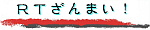\$B8=:_\$N>l=j(B :
 [\$B
English
\$B%Z!<%8<9I.(B 2002. 10. 2
\$B:G=*99?7(B 2002. 10. 2

# DTLR\$B\$N7zJ*(B\$B;#1FF|(B: 2002. 2. 9. (\$BBg\$-\$J2hA|(B)

\$B%m%s%I%s!&%t%#%/%H%j%"(B (Victoria) \$B1X\$=\$P\$K\$"\$k(B DTLR (Department of Transport, Local Government and the Regions) \$B\$N7zJ*!#(B \$BF|K\\$G\$\$\$(\$P9qEZ8rDL>J\$H\$\$\$C\$?\$H\$3\$m\$+\$H;W\$o\$l\$^\$9!#(B \$B0lEY!"(B \$B\$3\$N7zJ*Fb\$G%W%l%<%s%F!<%7%g%s\$r\$7\$?\$3\$H\$,\$"\$j\$^\$9!#(B

\$B2hA|\$KCx:n8"I=<(\$NJ8;zNs\$,\$G\$k>l9g!"(B \$B\$3\$A\$i\$r%/%j%C%/!#(B
\$B9bLZ(B \$BN<(B webmaster@takagi-ryo.ac